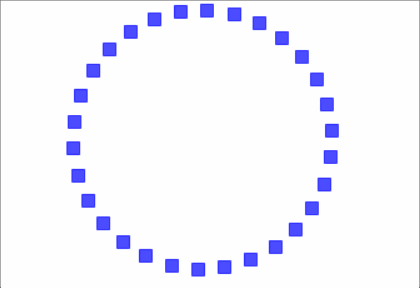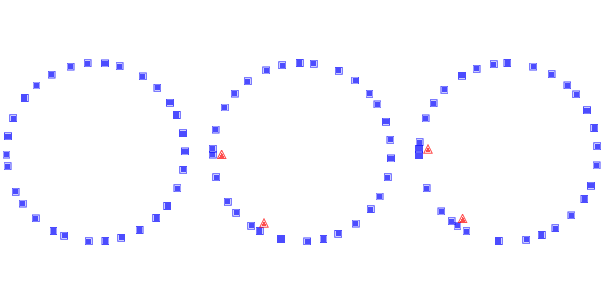# 12.2. Traffic Jams¶Gif 12.1: Simulation of traffic jams. Squares indicate the position of the drivers; triangles indicate places where one driver has to brake to avoid another.

What causes traffic jams? Sometimes there is an obvious cause, like an accident, a speed trap, or something else that disturbs the flow of traffic. But other times traffic jams appear for no apparent reason.

Agent-based models can help explain spontaneous traffic jams. As an example, we implement a highway simulation based on a model in Mitchell Resnick’s book, Turtles, Termites and Traffic Jams.

Here’s the class that represents the “highway”:

```class Highway:

def __init__(self, n=10, length=1000, eps=0):
self.length = length
self.eps = eps

# create the drivers
locs = np.linspace(0, length, n, endpoint=False)
self.drivers = [Driver(loc) for loc in locs]

for i in range(n):
j = (i+1) % n
self.drivers[i].next = self.drivers[j]
```

`n` is the number of cars, `length` is the length of the highway, and `eps` is the amount of random noise we’ll add to the system.

`locs` contains the locations of the drivers; the NumPy function `linspace` creates an array of `n` locations equally spaced between `0` and `length`.

The `drivers` attribute is a list of `Driver` objects. The for loop links them so each `Driver` contains a reference to the next. The highway is circular, so the last `Driver` contains a reference to the first.

During each time step, the `Highway` moves each of the `drivers`:

```# Highway

def step(self):
for driver in self.drivers:
self.move(driver)
```

The move method lets the `Driver` choose its acceleration. Then `move` computes the updated `speed` and position. Here’s the implementation:

```# Highway

def move(self, driver):
dist = self.distance(driver)

# let the driver choose acceleration
acc = driver.choose_acceleration(dist)
acc = min(acc, self.max_acc)
acc = max(acc, self.min_acc)
speed = driver.speed + acc

# add random noise to speed
speed *= np.random.uniform(1-self.eps, 1+self.eps)

# keep it nonnegative and under the speed limit
speed = max(speed, 0)
speed = min(speed, self.speed_limit)

# if current speed would collide, stop
if speed > dist:
speed = 0

# update speed and loc
driver.speed = speed
driver.loc += speed
```

`dist` is the distance between `driver` and the next `driver` ahead. This distance is passed to `choose_acceleration`, which specifies the behavior of the driver. This is the only decision the driver gets to make; everything else is determined by the “physics” of the simulation.

• `acc` is acceleration, which is bounded by `min_acc` and `max_acc`. In the given implementation, cars can accelerate with `max_acc=1` and brake with `min_acc=-10`.

• `speed` is the old speed plus the requested acceleration, but then we make some adjustments. First, we add random noise to `speed`, because the world is not perfect. `eps` determines the magnitude of the relative error; for example, if `eps` is 0.02, `speed` is multiplied by a random factor between 0.98 and 1.02.

• Speed is bounded between 0 and `speed_limit`, which is 40 in the given implementation, so cars are not allowed to go backward or speed.

• If the requested speed would cause a collision with the next car, `speed` is set to 0.

• Finally, we update the `speed` and `loc` attributes of `driver`.

Here’s the definition for the `Driver` class:

```class Driver:

def __init__(self, loc, speed=0):
self.loc = loc
self.speed = speed

def choose_acceleration(self, dist):
return 1
```

The attributes `loc` and `speed` are the location and speed of the driver.

This implementation of `choose_acceleration` is simple: it always accelerates at the maximum rate.

Since the cars start out equally spaced, we expect them all to accelerate until they reach the speed limit, or until their speed exceeds the space between them. At that point, at least one “collision” will occur, causing some cars to stop.Figure 12.1: Simulation of drivers on a circular highway at three points in time. Squares indicate the position of the drivers; triangles indicate places where one driver has to brake to avoid another.

Figure 12.1 shows a few steps in this process, starting with 30 cars and `eps=0.02`. On the left is the configuration after 16 time steps, with the highway mapped to a circle. Because of random noise, some cars are going faster than others, and the spacing has become uneven.

During the next time step (middle) there are two collisions, indicated by the triangles.

During the next time step (right) two cars collide with the stopped cars, and we can see the initial formation of a traffic jam. Once a jam forms, it tends to persist, with additional cars approaching from behind and colliding, and with cars in the front accelerating away.

Under some conditions, the jam itself propagates backwards, as you can see if you watch the animations in the notebook for this chapter.